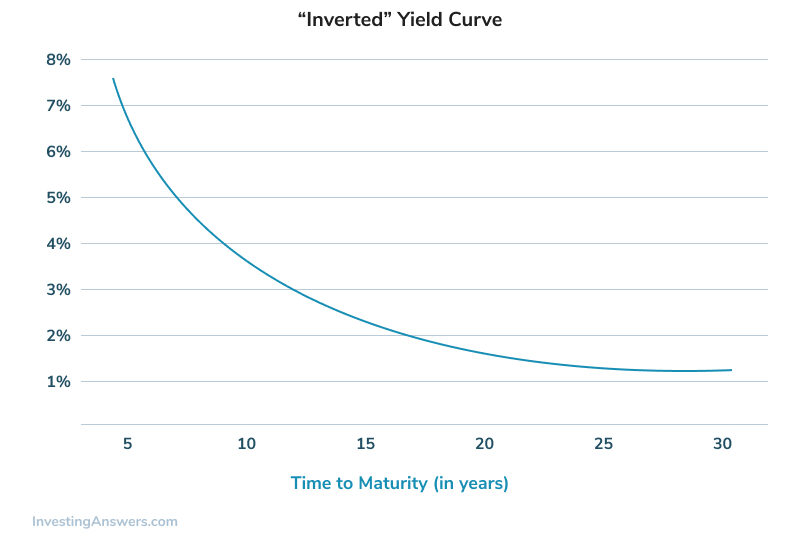## What is an Inverted Yield Curve?

An inverted yield curve, also called a negative yield curve, is a yield curve indicating that short-term yields are higher than long-term yields.

## How Does the Yield Curve Work? (Chart and Example)

Also known as the term structure of interest rates, the yield curve is a graph that plots the yields of similar-quality bonds against their maturities, ranging from shortest to longest. Note that the chart does not plot coupon rates against a range of maturities -- that's called a spot curve.

There are two other types of yield curves. If short-term yields are lower than long-term yields, the curve is called a positive (or 'normal') yield curve. A flat yield curve exists when there is little or no difference between short- and long-term yields. See the picture below for an example of an inverted yield curve.The shape of any yield curve changes over time, and yield curves are calculated and published by The Wall Street Journal, the Federal Reserve and many financial institutions.

## Why Does an Inverted Yield Curve Predict a Recession?

Because the yield curve is generally indicative of future interest rates, which follow an economy's expansion or contraction, yield curves and changes in yield curves can convey much information.

Generally, an inverted yield curve indicates that investors require a higher rate of return for taking the added risk of lending money for a shorter period of time. Many economists also believe that a steep positive curve indicates investors expect higher future inflation (and thus higher interest rates), and that a sharply inverted yield curve means investors expect lower inflation (and interest rates) in the future.

A flattening yield curve generally indicates investors are unsure about future economic growth.

In the 1990s, Duke University professor Campbell Harvey found that inverted yield curves have preceded the last five U.S. recessions. Changes in the shape of the yield curve also affect a portfolio's return in that they make some bonds more or less valuable relative to other bonds. These concepts are part of what motivates analysts to study yield curves carefully.